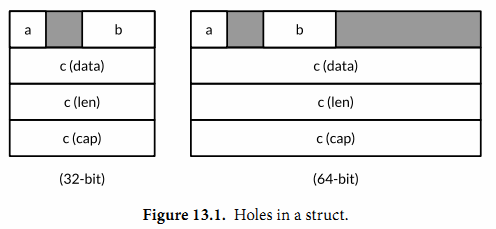## 13.1. unsafe.Sizeof, Alignof 和 Offsetof

unsafe.Sizeof函數返迴操作數在內存中的字節大小，參數可以是任意類型的表達式，但是它併不會對表達式進行求值。一個Sizeof函數調用是一個對應uintptr類型的常量表達式，因此返迴的結果可以用作數組類型的長度大小，或者用作計算其他的常量。

import "unsafe"
fmt.Println(unsafe.Sizeof(float64(0))) // "8"


Sizeof函數返迴的大小隻包括數據結構中固定的部分，例如字符串對應結構體中的指針和字符串長度部分，但是併不包含指針指向的字符串的內容。Go語言中非聚合類型通常有一個固定的大小，盡管在不同工具鏈下生成的實際大小可能會有所不同。考慮到可移植性，引用類型或包含引用類型的大小在32位平台上是4個字節，在64位平台上是8個字節。

bool 1個字節
intN, uintN, floatN, complexN N/8個字節(例如float64是8個字節)
int, uint, uintptr 1個機器字
*T 1個機器字
string 2個機器字(data,len)
[]T 3個機器字(data,len,cap)
map 1個機器字
func 1個機器字
chan 1個機器字
interface 2個機器字(type,value)

Go語言的規范併沒有要求一個字段的聲明順序和內存中的順序是一致的，所以理論上一個編譯器可以隨意地重新排列每個字段的內存位置，隨然在寫作本書的時候編譯器還沒有這麽做。下面的三個結構體雖然有着相同的字段，但是第一種寫法比另外的兩個需要多50%的內存。

                               // 64-bit  32-bit
struct{ bool; float64; int16 } // 3 words 4words
struct{ float64; int16; bool } // 2 words 3words
struct{ bool; int16; float64 } // 2 words 3words


unsafe.Alignof 函數返迴對應參數的類型需要對齊的倍數. 和 Sizeof 類似, Alignof 也是返迴一個常量表達式, 對應一個常量. 通常情況下布爾和數字類型需要對齊到它們本身的大小(最多8個字節), 其它的類型對齊到機器字大小.

unsafe.Offsetof 函數的參數必鬚是一個字段 x.f, 然後返迴 f 字段相對於 x 起始地址的偏移量, 包括可能的空洞.

var x struct {
a bool
b int16
c []int
}32位繫統：

Sizeof(x)   = 16  Alignof(x)   = 4
Sizeof(x.a) = 1   Alignof(x.a) = 1 Offsetof(x.a) = 0
Sizeof(x.b) = 2   Alignof(x.b) = 2 Offsetof(x.b) = 2
Sizeof(x.c) = 12  Alignof(x.c) = 4 Offsetof(x.c) = 4


64位繫統：

Sizeof(x)   = 32  Alignof(x)   = 8
Sizeof(x.a) = 1   Alignof(x.a) = 1 Offsetof(x.a) = 0
Sizeof(x.b) = 2   Alignof(x.b) = 2 Offsetof(x.b) = 2
Sizeof(x.c) = 24  Alignof(x.c) = 8 Offsetof(x.c) = 8